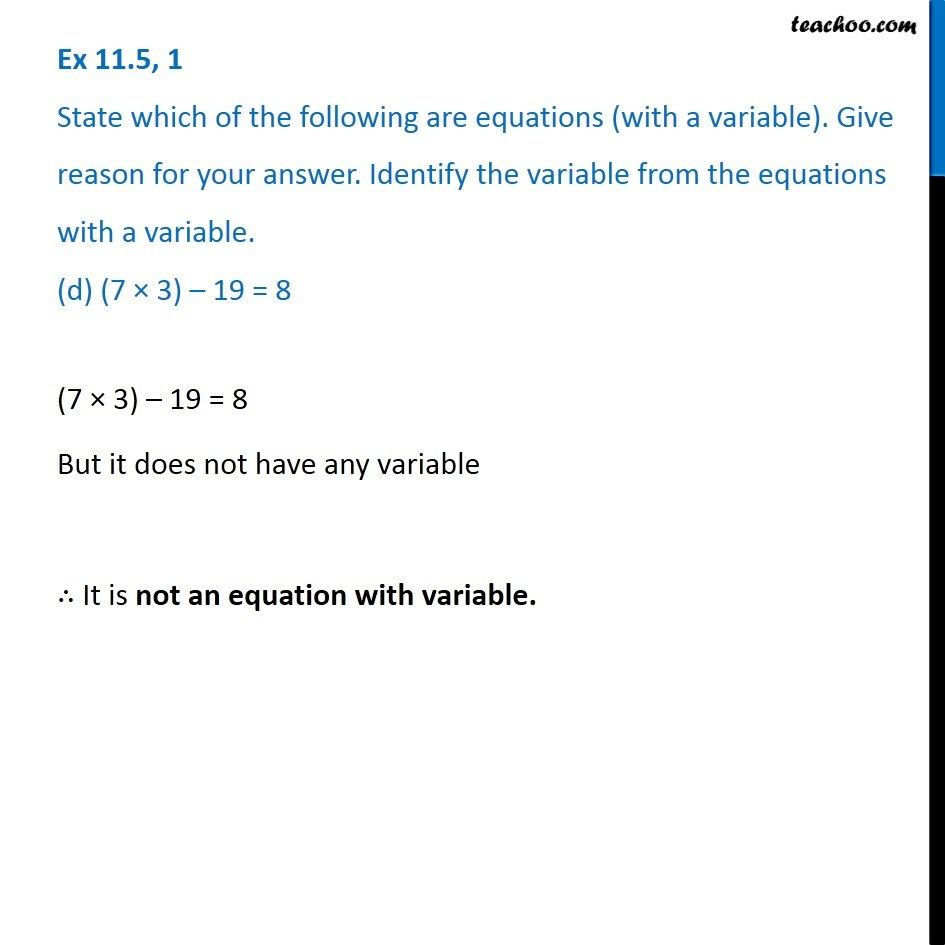Finding solutions of Algebra Equations - WorksheetLearn in your speed, with individual attention - Teachoo Maths 1-on-1 Class

### Transcript

Question 1 State which of the following are equations (with a variable). Give reason for your answer. Identify the variable from the equations with a variable. (d) (7 × 3) – 19 = 8(7 × 3) – 19 = 8 But it does not have any variable ∴ It is not an equation with variable.